# Methods and formulas for the model information in Analyze Factorial Design

## Design matrix

Minitab uses the same approach to the design matrix as used in general linear model (GLM), which uses regression to fit the model you specify. First Minitab creates a design matrix from the factors and the model that you specify. The columns of this matrix, called X, represent the terms in the model.

The design matrix has n rows, where n = number of observations and columns that correspond to the terms in the model. The columns for the terms have the following order in the design matrix:
1. Constant
2. Covariates
3. Blocks
4. Factors
5. Interactions
These types of terms have 1 column each in the design matrix:
• Constant
• Covariate
• Continuous factor

For blocks, the number of columns is one less than the number of blocks.

### Categorical factors and interactions in 2-level designs

In a 2-level design, the term for a categorical factor has 1 column. Any interaction terms also have 1 column.

### Categorical factors in general factorial designs

In a general factorial design, categorical factors can have multiple columns. The number of columns is 1 less than the number of levels. Suppose A is a factor with 4 levels. Then it has 3 degrees of freedom and its block contains 3 columns. Let these columns be A1, A2, A3. Each row is coded as one of the following:
Level of A A1 A2 A3
1 1 0 0
2 0 1 0
3 0 0 1
4 -1 -1 -1

### Interactions in general factorial designs

To calculate the columns for an interaction term, multiply the corresponding columns for the factors in the interaction. For example, suppose factor A has 6 levels, C has 3 levels, D has 4 levels. Then the term A * C * D has 5 x 2 x 3 = 30 columns. To obtain the levels, multiply each column for A by each for C, by each for D.

### Whole plot columns in split-plot designs

###### Note

Minitab does not analyze split-plot designs with a binary response.

For a split-plot design, Minitab uses 2 versions of the design matrix. One version is the same matrix used for any 2-level factorial design. The other matrix includes a block of columns that represent whole plots. Calculation, for example, of the whole plot error term uses this second version of the design matrix. The columns for whole plots follow the columns for the hard-to-change factors and interactions that involve only hard-to-change factors.

## Effects

Estimated effects for each factor. Effects are only calculated for two-level models and are not calculated for general factorial models. The formula for the effect of a factor is:

Effect = Coefficient * 2

## Coefficients (Coef)

The estimates of the population regression coefficients in a regression equation. For each factor, Minitab calculates k - 1 coefficients, where k is the number of levels in the factor. For a 2-factor, 2-level, full factorial model, the formulas for coefficients for the factors and interactions are:

The standard error of the coefficient for this 2-factor, 2-level, full factorial model is:

For information on models with more than two factors or factors with more than two levels, see Montgomery1.

### Notation

TermDescription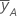mean of y at the high level of factor A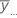overall mean of all observations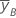mean of y at the high level of factor B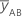mean of y at the high levels of A and B
MSEmean square error
nnumber of - 1's and 1's (in the covariance matrix) for the estimated term

## Box-Cox transformation

Box-Cox transformation selects lambda values, as shown below, which minimize the residual sum of squares. The resulting transformation is Y λ when λ ≠ 0 and ln(Y) when λ = 0. When λ < 0, Minitab also multiplies the transformed response by −1 to maintain the order from the untransformed response.

Minitab searches for an optimal value between −2 and 2. Values that fall outside of this interval might not result in a better fit.

Here are some common transformations where Y′ is the transform of the data Y:

Lambda (λ) value Transformation
λ = 2 Y′ = Y 2
λ = 0.5 Y′ =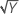λ = 0 Y′ = ln(Y )
λ = −0.5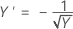λ = −1 Y′ = −1 / Y

## Weighted regression

Weighted least squares regression is a method for dealing with observations that have nonconstant variances. If the variances are not constant, observations with:

• large variances should be given relatively small weights
• small variances should be given relatively large weights

The usual choice of weights is the inverse of pure error variance in the response.

The formula for the estimated coefficients is as follows:
This is equivalent to minimizing the weighted SS Error.

### Notation

TermDescription
Xdesign matrix
X'transpose of the design matrix
Wan n x n matrix with the weights on the diagonal
Yvector of response values
nnumber of observations
wiweight for the ith observation
yiresponse value for the ith observationfitted value for the ith observation
1 D. C. Montgomery (1991) Design and Analysis of ExperimentsThird Edition, John Wiley & Sons.
By using this site you agree to the use of cookies for analytics and personalized content.  Read our policy